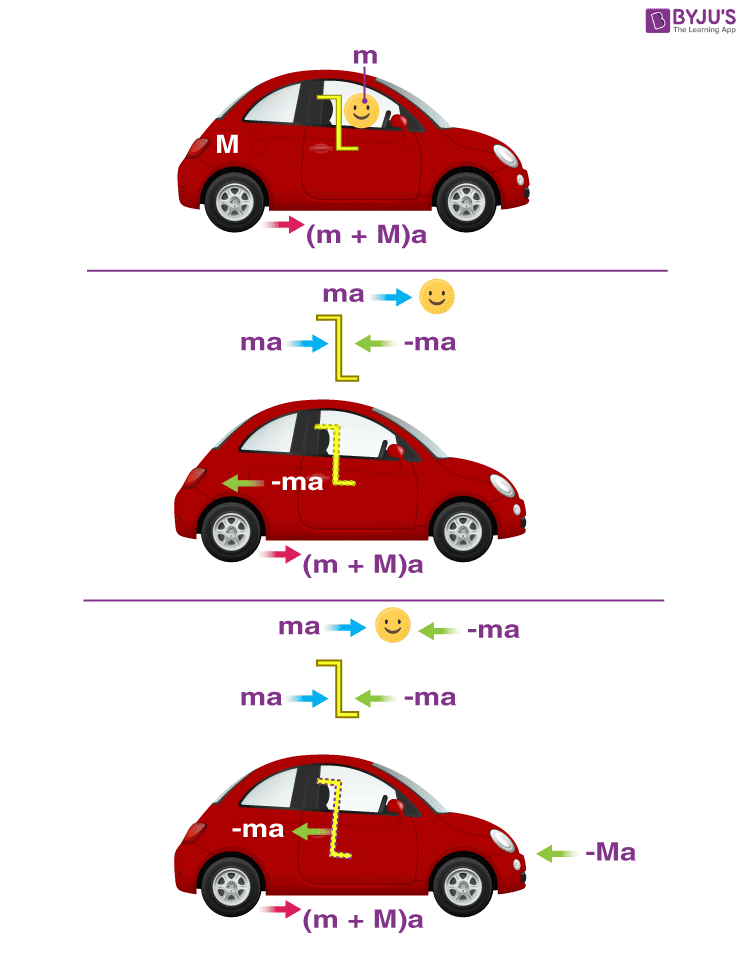# Pseudo Force

## What is Pseudo Force?

A Pseudo force (also called a fictitious force, inertial force or d’Alembert force) is an apparent force that acts on all masses whose motion is described using a non-inertial frame of reference, such as a rotating reference frame.

Pseudo force comes into effect when the frame of reference has started acceleration compared to a non-accelerating frame.

The force F does not arise from any physical interaction between two objects, but rather from the acceleration ‘a’ of the non-inertial reference frame itself. As a frame can accelerate in any arbitrary way, so can pseudo forces be arbitrary (but only in direct response to the acceleration of the frame). However, four pseudo forces are defined for frames accelerated in commonly occurring ways: one by a relative acceleration of the origin in a straight line (rectilinear acceleration); two involving rotation: Coriolis force and Centrifugal force and fourth called Euler force, caused by a variable rate of rotation.

### Examples of Pseudo Force:

For example, if you consider a person standing at a bus stop watching an accelerating car, he infers that a force is exerted on the car and it is accelerating. Here there is no problem and the pseudo force concept is not required

But, if the person inside the accelerating car is looking at the person standing at the bus stop, he finds that the person is accelerating with respect to the car, though no force is acting on it. Here, the concept of pseudo force is required to convert the non-inertial frame of reference to an equivalent inertial frame of reference.

For another example consider a ball hung from the roof of a train by means of an inextensible string. If the train is at rest or is moving with a uniform speed in a straight line the string will be vertical. A passenger will infer that the net force acting on the ball is zero.

If the train begins to accelerate, then the string will be making an angle with respect to the vertical. For the passenger, there are only two forces, and they are not collinear. But, the ball remains apparently in a state of equilibrium (as long as the acceleration of the train is constant). Here, the concept of pseudo force is required.## Figure 1:

### Top panel:

accelerating car of mass M with the passenger of mass m. The force from the axle is (m + M)a. In the inertial frame, this is the only force on the car and passenger.

### Centre panel:

an exploded view in the inertial frame. The passenger is subject to the accelerating force ma. The seat (assumed of negligible mass) is compressed between the reaction force –ma and the applied force from the car ma. The car is subject to the net acceleration force Ma which is the difference between the applied force (m + M)a from the axle and the reaction from the seat −ma.

### Bottom panel:

an exploded view in the non-inertial frame. In the non-inertial frame where the car is not accelerating, the force from the axle is balanced by a fictitious backward force −(m + M)a, a portion −Ma applied to the car, and −ma to the passenger. The car is subject to the fictitious force −Ma and the force (m + M)a from the axle. The difference between these forces ma is applied to the seat, which exerts a reaction −ma upon the car, so zero net force is applied to the car. The seat (assumed massless) transmits the force ma to the passenger, who is subject also to the fictitious force −ma, resulting in zero net force on the passenger. The passenger exerts a reaction force −ma upon the seat, which is therefore compressed. In all frames, the compression of the seat is the same, and the force delivered by the axle is the same

## Frequently Asked Questions – FAQs

Q1

### What is a pseudo force?

Suppose two bodies A and B are sitting in an accelerated bus and C observes both of them from the ground. So if C draws a free body diagram of B, it will have a net force that gives acceleration. But if A draws a free body diagram for B then for him B is at rest so to counter the force shown by A he needs a fictitious force in his frame. That force is known as a pseudo force.
Q2

### Is centripetal force always equal to centrifugal force?

This is not necessary. If we are in a frame of reference from where the body is at rest as long as the radial direction is concerned the two forces will be equal. But if there is radial acceleration in a body with respect to a frame then in that frame centripetal force will not be equal to centrifugal force.
Q3

### Is work done by these forces to be considered for the work-energy theorem?

When we are applying the work-energy theorem from a non-inertial frame of reference we need to take into account the work done by pseudo forces.
Q4

### How can these forces help in problem-solving?

Suppose we have a small block which is moving up a wedge that is accelerating with some acceleration. We need to find the time when the small block reaches the top of the wedge. Analysing this situation is much simpler if we are in the wedge frame of reference, in that case, the FBD will have an additional pseudo force as the wedge is accelerated.

## Watch the video and understand Coriolis Effect in detail.